Скачать презентацию Aswath Damodaran OPTION PRICING BASICS Aswath Damodaran 1

950868a3aaaebb0326cfa76c26a22b4e.ppt

• Количество слайдов: 16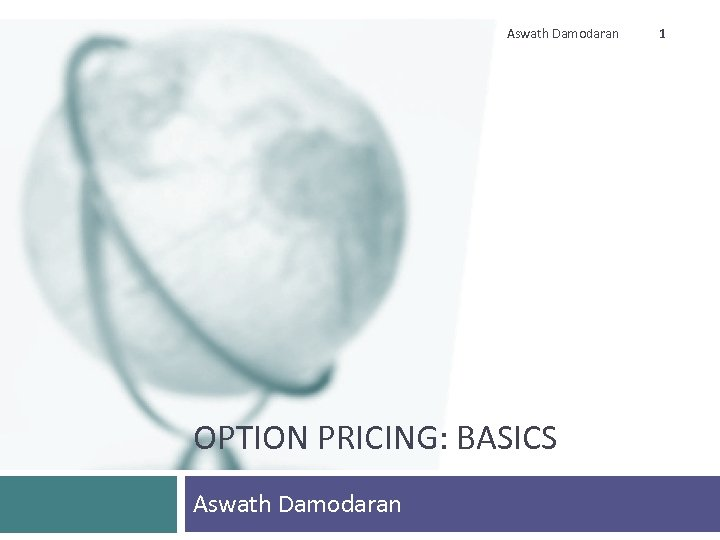Aswath Damodaran OPTION PRICING: BASICS Aswath Damodaran 1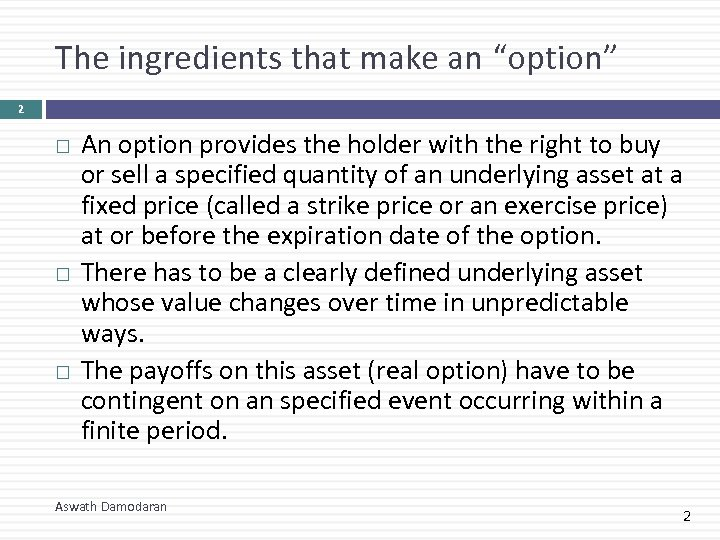The ingredients that make an “option” 2 An option provides the holder with the right to buy or sell a specified quantity of an underlying asset at a fixed price (called a strike price or an exercise price) at or before the expiration date of the option. There has to be a clearly defined underlying asset whose value changes over time in unpredictable ways. The payoffs on this asset (real option) have to be contingent on an specified event occurring within a finite period. Aswath Damodaran 2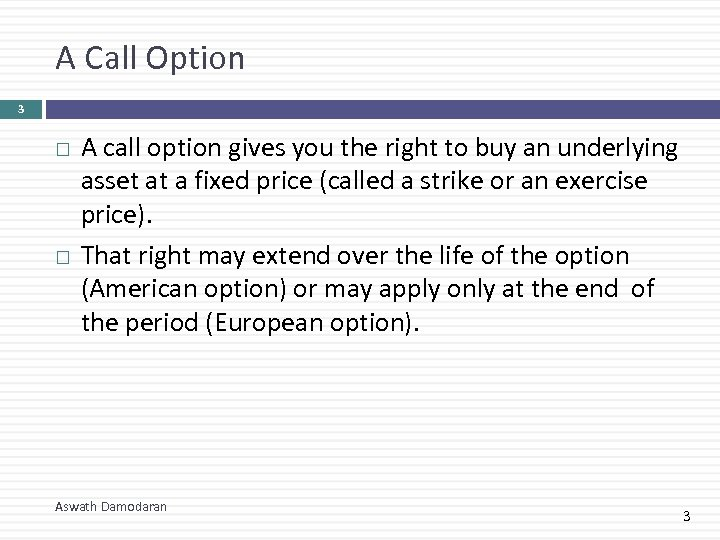A Call Option 3 A call option gives you the right to buy an underlying asset at a fixed price (called a strike or an exercise price). That right may extend over the life of the option (American option) or may apply only at the end of the period (European option). Aswath Damodaran 3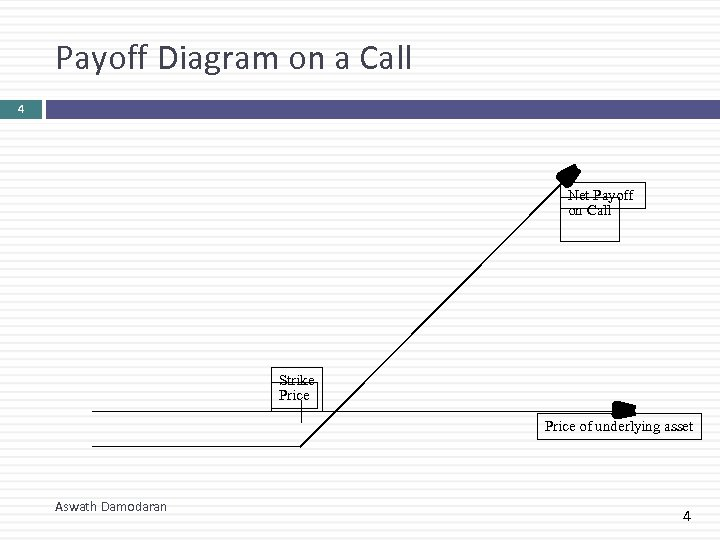Payoff Diagram on a Call 4 Net Payoff on Call Strike Price of underlying asset Aswath Damodaran 4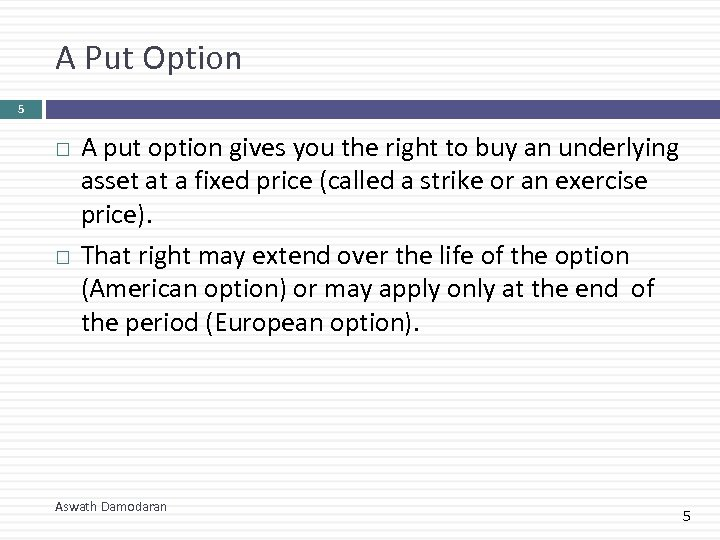A Put Option 5 A put option gives you the right to buy an underlying asset at a fixed price (called a strike or an exercise price). That right may extend over the life of the option (American option) or may apply only at the end of the period (European option). Aswath Damodaran 5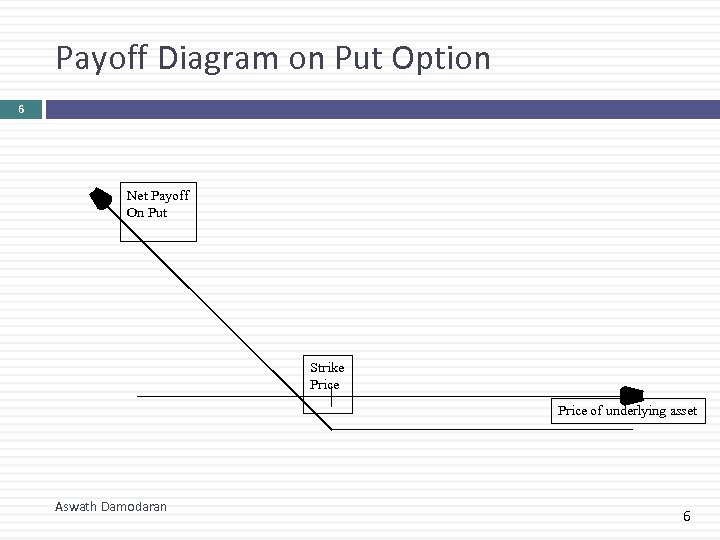Payoff Diagram on Put Option 6 Net Payoff On Put Strike Price of underlying asset Aswath Damodaran 6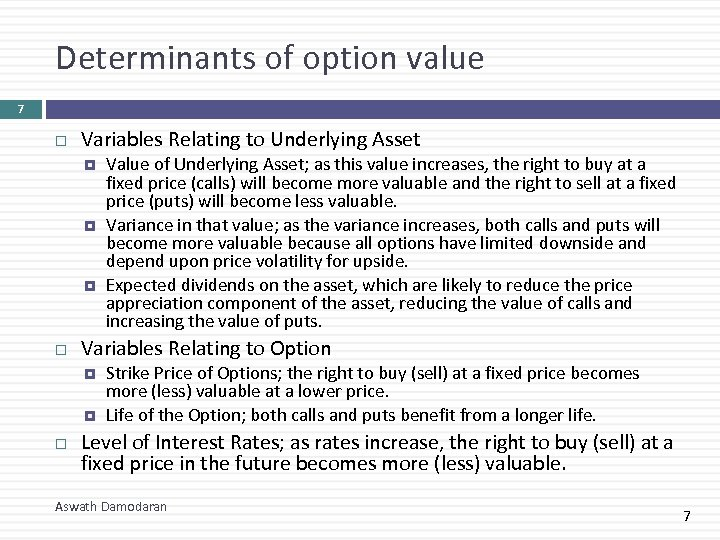Determinants of option value 7 Variables Relating to Underlying Asset Variables Relating to Option Value of Underlying Asset; as this value increases, the right to buy at a fixed price (calls) will become more valuable and the right to sell at a fixed price (puts) will become less valuable. Variance in that value; as the variance increases, both calls and puts will become more valuable because all options have limited downside and depend upon price volatility for upside. Expected dividends on the asset, which are likely to reduce the price appreciation component of the asset, reducing the value of calls and increasing the value of puts. Strike Price of Options; the right to buy (sell) at a fixed price becomes more (less) valuable at a lower price. Life of the Option; both calls and puts benefit from a longer life. Level of Interest Rates; as rates increase, the right to buy (sell) at a fixed price in the future becomes more (less) valuable. Aswath Damodaran 7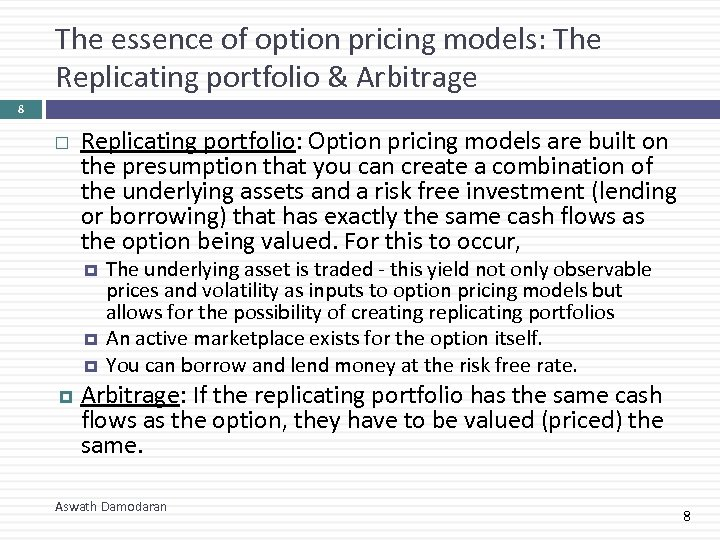The essence of option pricing models: The Replicating portfolio & Arbitrage 8 Replicating portfolio: Option pricing models are built on the presumption that you can create a combination of the underlying assets and a risk free investment (lending or borrowing) that has exactly the same cash flows as the option being valued. For this to occur, The underlying asset is traded - this yield not only observable prices and volatility as inputs to option pricing models but allows for the possibility of creating replicating portfolios An active marketplace exists for the option itself. You can borrow and lend money at the risk free rate. Arbitrage: If the replicating portfolio has the same cash flows as the option, they have to be valued (priced) the same. Aswath Damodaran 8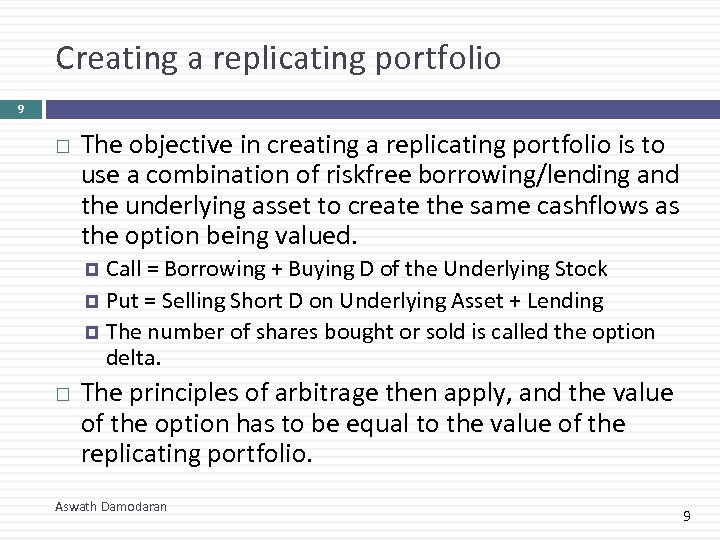Creating a replicating portfolio 9 The objective in creating a replicating portfolio is to use a combination of riskfree borrowing/lending and the underlying asset to create the same cashflows as the option being valued. Call = Borrowing + Buying D of the Underlying Stock Put = Selling Short D on Underlying Asset + Lending The number of shares bought or sold is called the option delta. The principles of arbitrage then apply, and the value of the option has to be equal to the value of the replicating portfolio. Aswath Damodaran 9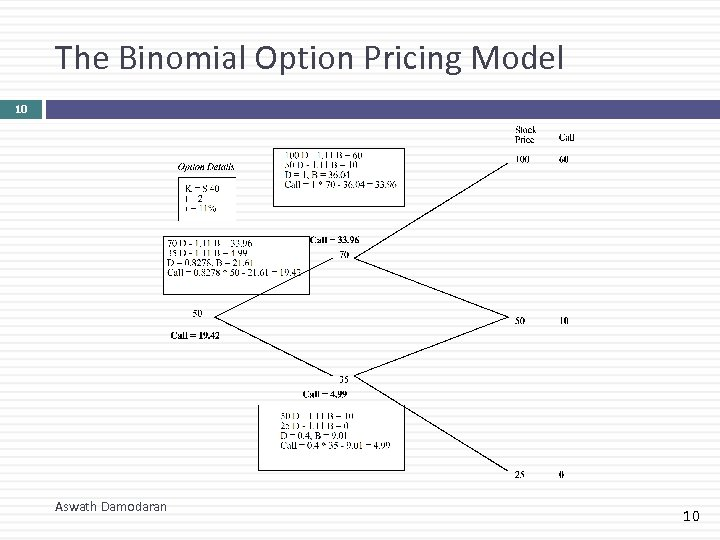The Binomial Option Pricing Model 10 Aswath Damodaran 10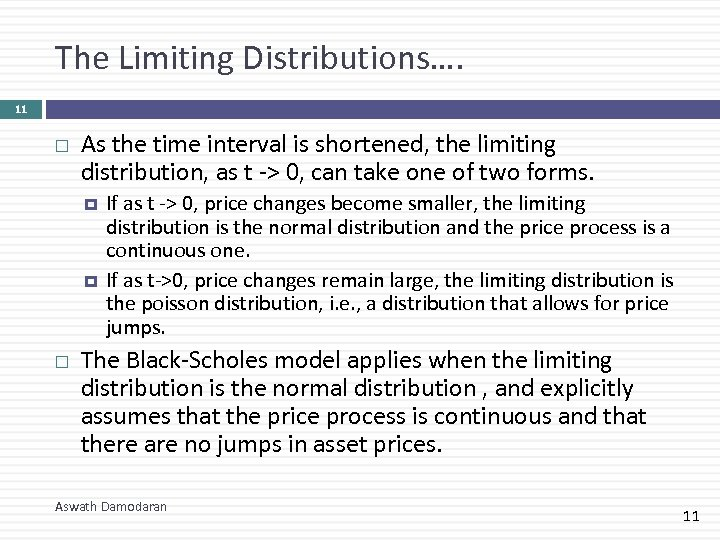The Limiting Distributions…. 11 As the time interval is shortened, the limiting distribution, as t -> 0, can take one of two forms. If as t -> 0, price changes become smaller, the limiting distribution is the normal distribution and the price process is a continuous one. If as t->0, price changes remain large, the limiting distribution is the poisson distribution, i. e. , a distribution that allows for price jumps. The Black-Scholes model applies when the limiting distribution is the normal distribution , and explicitly assumes that the price process is continuous and that there are no jumps in asset prices. Aswath Damodaran 11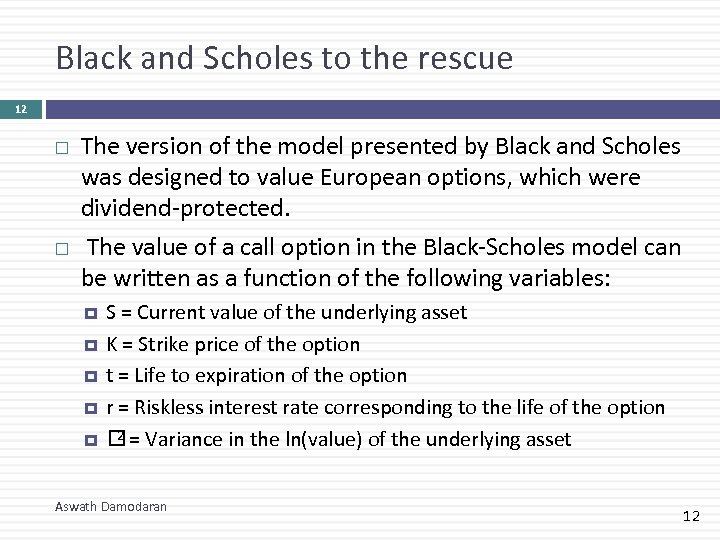Black and Scholes to the rescue 12 The version of the model presented by Black and Scholes was designed to value European options, which were dividend-protected. The value of a call option in the Black-Scholes model can be written as a function of the following variables: S = Current value of the underlying asset K = Strike price of the option t = Life to expiration of the option r = Riskless interest rate corresponding to the life of the option 2 = Variance in the ln(value) of the underlying asset Aswath Damodaran 12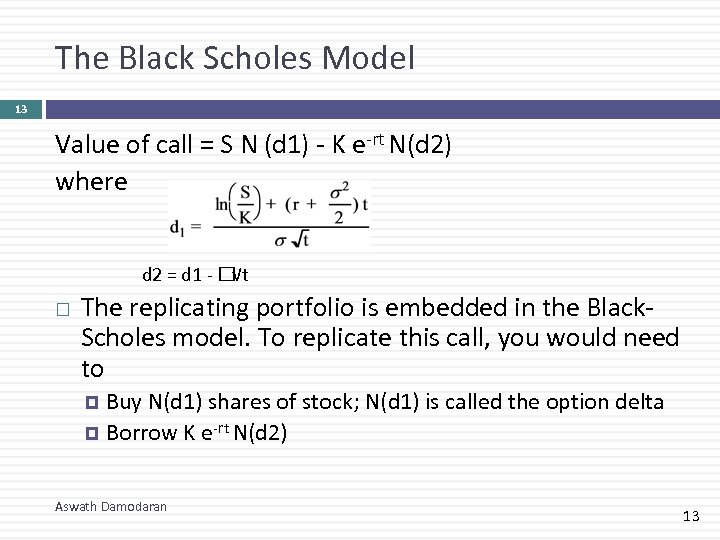The Black Scholes Model 13 Value of call = S N (d 1) - K e-rt N(d 2) where d 2 = d 1 - √t The replicating portfolio is embedded in the Black. Scholes model. To replicate this call, you would need to Buy N(d 1) shares of stock; N(d 1) is called the option delta Borrow K e-rt N(d 2) Aswath Damodaran 13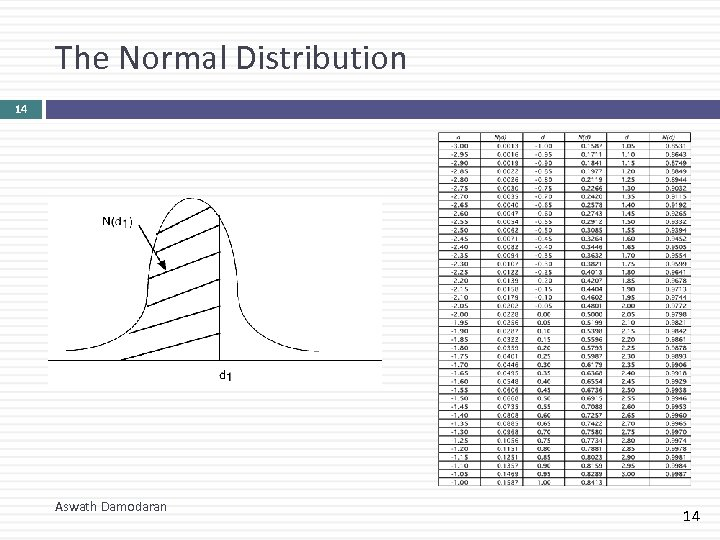The Normal Distribution 14 Aswath Damodaran 14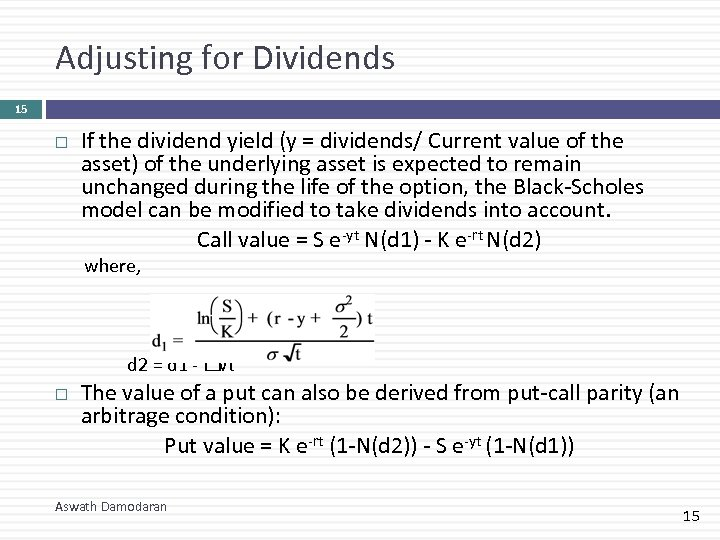Adjusting for Dividends 15 If the dividend yield (y = dividends/ Current value of the asset) of the underlying asset is expected to remain unchanged during the life of the option, the Black-Scholes model can be modified to take dividends into account. Call value = S e-yt N(d 1) - K e-rt N(d 2) where, d 2 = d 1 - √t The value of a put can also be derived from put-call parity (an arbitrage condition): Put value = K e-rt (1 -N(d 2)) - S e-yt (1 -N(d 1)) Aswath Damodaran 15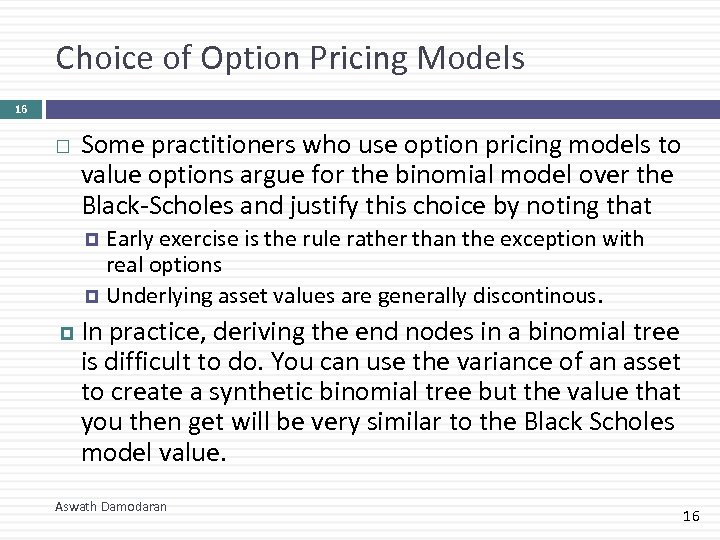Choice of Option Pricing Models 16 Some practitioners who use option pricing models to value options argue for the binomial model over the Black-Scholes and justify this choice by noting that Early exercise is the rule rather than the exception with real options Underlying asset values are generally discontinous. In practice, deriving the end nodes in a binomial tree is difficult to do. You can use the variance of an asset to create a synthetic binomial tree but the value that you then get will be very similar to the Black Scholes model value. Aswath Damodaran 16